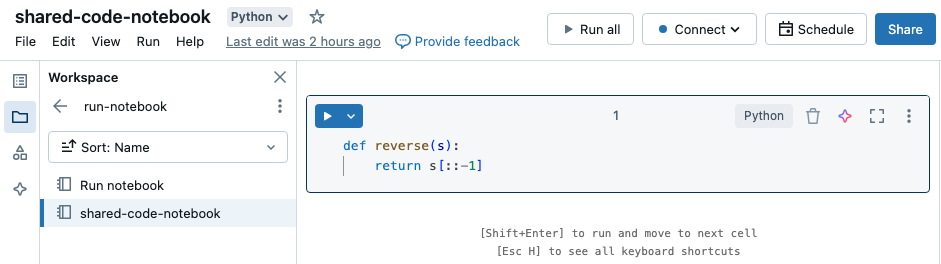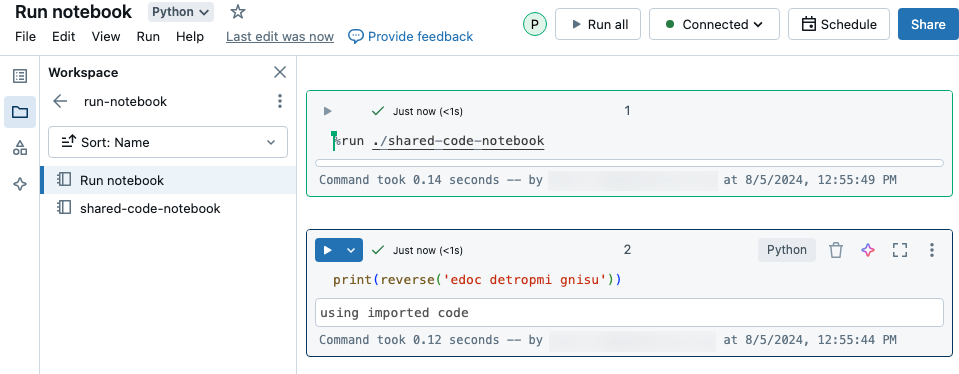# Run a Databricks notebook from another notebook

Note

For most orchestration use cases, Databricks recommends using Databricks Jobs or modularizing your code with files. You should only use the `dbutils.notebook` API described in this article when your use case cannot be implemented using multi-task jobs. Examples are conditional execution and looping notebooks over a dynamic set of parameters.

This article describes how to use Databricks notebooks to code complex workflows that use modular code, linked or embedded notebooks, and if-then-else logic.

## Comparison of `%run` and `dbutils.notebook.run()`

The %run command allows you to include another notebook within a notebook. You can use `%run` to modularize your code, for example by putting supporting functions in a separate notebook. You can also use it to concatenate notebooks that implement the steps in an analysis. When you use `%run`, the called notebook is immediately executed and the functions and variables defined in it become available in the calling notebook.

The `dbutils.notebook` API is a complement to `%run` because it lets you pass parameters to and return values from a notebook. This allows you to build complex workflows and pipelines with dependencies. For example, you can get a list of files in a directory and pass the names to another notebook, which is not possible with `%run`. You can also create if-then-else workflows based on return values or call other notebooks using relative paths.

Unlike `%run`, the `dbutils.notebook.run()` method starts a new job to run the notebook.

These methods, like all of the `dbutils` APIs, are available only in Python and Scala. However, you can use `dbutils.notebook.run()` to invoke an R notebook.

## Use `%run` to import a notebook

In this example, the first notebook defines a function, `reverse`, which is available in the second notebook after you use the `%run` magic to execute `shared-code-notebook`.Because both of these notebooks are in the same directory in the workspace, use the prefix `./` in `./shared-code-notebook` to indicate that the path should be resolved relative to the currently running notebook. You can organize notebooks into directories, such as `%run ./dir/notebook`, or use an absolute path like `%run /Users/username@organization.com/directory/notebook`.

Note

• `%run` must be in a cell by itself, because it runs the entire notebook inline.

• You cannot use `%run` to run a Python file and `import` the entities defined in that file into a notebook. To import from a Python file, see Modularize your code using files. Or, package the file into a Python library, create a Databricks library from that Python library, and install the library into the cluster you use to run your notebook.

• When you use `%run` to run a notebook that contains widgets, by default the specified notebook runs with the widget’s default values. You can also pass in values to widgets; see Use Databricks widgets with %run.

## `dbutils.notebook` API

The methods available in the `dbutils.notebook` API are `run` and `exit`. Both parameters and return values must be strings.

`run(path: String,  timeout_seconds: int, arguments: Map): String`

Run a notebook and return its exit value. The method starts an ephemeral job that runs immediately.

The `timeout_seconds` parameter controls the timeout of the run (0 means no timeout): the call to `run` throws an exception if it doesn’t finish within the specified time. If Databricks is down for more than 10 minutes, the notebook run fails regardless of `timeout_seconds`.

The `arguments` parameter sets widget values of the target notebook. Specifically, if the notebook you are running has a widget named `A`, and you pass a key-value pair `("A": "B")` as part of the arguments parameter to the `run()` call, then retrieving the value of widget `A` will return `"B"`. You can find the instructions for creating and working with widgets in the Databricks widgets article.

Note

• The `arguments` parameter accepts only Latin characters (ASCII character set). Using non-ASCII characters returns an error.

• Jobs created using the `dbutils.notebook` API must complete in 30 days or less.

### `run` Usage

```dbutils.notebook.run("notebook-name", 60, {"argument": "data", "argument2": "data2", ...})
```
```dbutils.notebook.run("notebook-name", 60, Map("argument" -> "data", "argument2" -> "data2", ...))
```

### `run` Example

Suppose you have a notebook named `workflows` with a widget named `foo` that prints the widget’s value:

```dbutils.widgets.text("foo", "fooDefault", "fooEmptyLabel")
print dbutils.widgets.get("foo")
```

Running `dbutils.notebook.run("workflows", 60, {"foo": "bar"})` produces the following result:The widget had the value you passed in using `dbutils.notebook.run()`, `"bar"`, rather than the default.

`exit(value: String): void` Exit a notebook with a value. If you call a notebook using the `run` method, this is the value returned.

```dbutils.notebook.exit("returnValue")
```

Calling `dbutils.notebook.exit` in a job causes the notebook to complete successfully. If you want to cause the job to fail, throw an exception.

## Example

In the following example, you pass arguments to `DataImportNotebook` and run different notebooks (`DataCleaningNotebook` or `ErrorHandlingNotebook`) based on the result from `DataImportNotebook`.When the code runs, you see a link to the running notebook:To view the details of the run, click the notebook link Notebook job #xxxx.## Pass structured data

This section illustrates how to pass structured data between notebooks.

```# Example 1 - returning data through temporary views.
# You can only return one string using dbutils.notebook.exit(), but since called notebooks reside in the same JVM, you can
# return a name referencing data stored in a temporary view.

## In callee notebook
spark.range(5).toDF("value").createOrReplaceGlobalTempView("my_data")
dbutils.notebook.exit("my_data")

## In caller notebook
returned_table = dbutils.notebook.run("LOCATION_OF_CALLEE_NOTEBOOK", 60)
global_temp_db = spark.conf.get("spark.sql.globalTempDatabase")
display(table(global_temp_db + "." + returned_table))

# Example 2 - returning data through DBFS.
# For larger datasets, you can write the results to DBFS and then return the DBFS path of the stored data.

## In callee notebook
dbutils.fs.rm("/tmp/results/my_data", recurse=True)
dbutils.notebook.exit("dbfs:/tmp/results/my_data")

## In caller notebook
returned_table = dbutils.notebook.run("LOCATION_OF_CALLEE_NOTEBOOK", 60)

# Example 3 - returning JSON data.
# To return multiple values, you can use standard JSON libraries to serialize and deserialize results.

## In callee notebook
import json
dbutils.notebook.exit(json.dumps({
"status": "OK",
"table": "my_data"
}))

## In caller notebook
result = dbutils.notebook.run("LOCATION_OF_CALLEE_NOTEBOOK", 60)
```
```// Example 1 - returning data through temporary views.
// You can only return one string using dbutils.notebook.exit(), but since called notebooks reside in the same JVM, you can
// return a name referencing data stored in a temporary view.

/** In callee notebook */
sc.parallelize(1 to 5).toDF().createOrReplaceGlobalTempView("my_data")
dbutils.notebook.exit("my_data")

/** In caller notebook */
val returned_table = dbutils.notebook.run("LOCATION_OF_CALLEE_NOTEBOOK", 60)
val global_temp_db = spark.conf.get("spark.sql.globalTempDatabase")
display(table(global_temp_db + "." + returned_table))

// Example 2 - returning data through DBFS.
// For larger datasets, you can write the results to DBFS and then return the DBFS path of the stored data.

/** In callee notebook */
dbutils.fs.rm("/tmp/results/my_data", recurse=true)
sc.parallelize(1 to 5).toDF().write.format("parquet").save("dbfs:/tmp/results/my_data")
dbutils.notebook.exit("dbfs:/tmp/results/my_data")

/** In caller notebook */
val returned_table = dbutils.notebook.run("LOCATION_OF_CALLEE_NOTEBOOK", 60)

// Example 3 - returning JSON data.
// To return multiple values, you can use standard JSON libraries to serialize and deserialize results.

/** In callee notebook */

// Import jackson json libraries
import com.fasterxml.jackson.module.scala.DefaultScalaModule
import com.fasterxml.jackson.module.scala.experimental.ScalaObjectMapper
import com.fasterxml.jackson.databind.ObjectMapper

// Create a json serializer
val jsonMapper = new ObjectMapper with ScalaObjectMapper
jsonMapper.registerModule(DefaultScalaModule)

// Exit with json
dbutils.notebook.exit(jsonMapper.writeValueAsString(Map("status" -> "OK", "table" -> "my_data")))

/** In caller notebook */
val result = dbutils.notebook.run("LOCATION_OF_CALLEE_NOTEBOOK", 60)
```

## Handle errors

This section illustrates how to handle errors.

```# Errors throw a WorkflowException.

def run_with_retry(notebook, timeout, args = {}, max_retries = 3):
num_retries = 0
while True:
try:
return dbutils.notebook.run(notebook, timeout, args)
except Exception as e:
if num_retries > max_retries:
raise e
else:
print("Retrying error", e)
num_retries += 1

run_with_retry("LOCATION_OF_CALLEE_NOTEBOOK", 60, max_retries = 5)
```
```// Errors throw a WorkflowException.

import com.databricks.WorkflowException

// Since dbutils.notebook.run() is just a function call, you can retry failures using standard Scala try-catch
// control flow. Here we show an example of retrying a notebook a number of times.
def runRetry(notebook: String, timeout: Int, args: Map[String, String] = Map.empty, maxTries: Int = 3): String = {
var numTries = 0
while (true) {
try {
return dbutils.notebook.run(notebook, timeout, args)
} catch {
case e: WorkflowException if numTries < maxTries =>
println("Error, retrying: " + e)
}
numTries += 1
}
"" // not reached
}

runRetry("LOCATION_OF_CALLEE_NOTEBOOK", timeout = 60, maxTries = 5)
```

## Run multiple notebooks concurrently

You can run multiple notebooks at the same time by using standard Scala and Python constructs such as Threads (Scala, Python) and Futures (Scala, Python). The example notebooks demonstrate how to use these constructs. To run the example: